# Arithmetic Coding and the 1MB Sorting Problem

It’s been two weeks since the 1MB Sorting Problem was originally featured on Reddit, and in case you don’t think this artificial problem has been thoroughly stomped into the ground yet, here’s a continuation of last post’s explanation of the working C++ program which solves it.

In that post, I gave a high-level outline of the approach, and showed an encoding scheme – which I’ve since learned is Golomb coding – which comes close to meeting the memory requirements, but doesn’t quite fit. Arithmetic coding, on the other hand, does fit. It’s interesting, because this problem, as it was phrased, almost seems designed to force you into arithmetic coding (though Nick Cleaton’s solution manages to avoid it).

I had read about arithmetic coding before, but I never had any reason to sit down to implement it. It always struck me as kind of mystical: How the heck you encode information in a fraction of a bit, anyway? The 1MB Sorting Problem turned out to be a great excuse to learn how arithmetic coding works.

## How Many Sorted Sequences Even Exist?

It’s important to note that the whole reason why we are able to represent a sorted sequence of one million 8-digit numbers in less than 1 MB of memory is because mathematically, there simply aren’t that many different sorted sequences which exist.

To see this, consider the following method of encoding a sorted sequence as a row of boxes, read from left to right.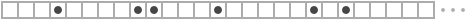An empty box tells us to increment the current value (initially 0), and a box with a dot inside it tells us to return the current value as part of the sorted sequence. For example, the above row of boxes corresponds to the sorted sequence { 3, 7, 7, 10, 15, 16, … }.

Now, to encode one million 8-digit numbers, we’d need exactly 1000000 boxes containing dots, plus a maximum of 99999999 empty boxes. In fact, when there are exactly 99999999 + 1000000 boxes in total, we can encode every possible sorted sequence using a unique distribution of dots. The question then becomes: How many different ways are there to distribute those dots? This is a straightforward number of combinations problem:

That’s a lot of combinations. Now, think of the contents of memory as one giant label which represents exactly one of those combinations. The exponent on the 2, above, gives us a lower limit for how many bits of memory would be required to come up with a unique label for every possible combination. In this case, it can’t be done using fewer than 8093730 bits, or 1011717 bytes.

That’s the fundamental limit. No encoding scheme can ever do better than that; it would be like trying to uniquely label every state in the USA using fewer than 6 bits. On the bright side, 1011717 bytes is comfortably less than our 1048576 byte limit, which is encouraging.

## The Probability of Encountering Each Delta Value

In the last post, we saw the potential of encoding delta values – the differences between numbers in a sorted sequence. Thinking in terms of the above rows of boxes, let’s take a look at the probability of encountering each delta value.

Since we know that there are 99999999 empty boxes, and 1000000 boxes containing dots, the probability of any particular box containing a dot is just:

For simplicity, let’s now imagine an infinite row of boxes, with dots occurring at the same frequency as this. The probability of encountering a delta value of 0 is, then, the same as the probability of a box containing a dot, which is just D.

How about a delta value of 1? Well, the probability of the first box being empty is (1 - D), while the probability of the second box containing a dot is still just D. Since each outcome is an independent event, we can multiply those probabilities together. The probability of encountering a delta value of 1, then, is

And in general, the probability encountering a delta value of N is

Now, let’s draw the real number line in the interval [0, 1), and let’s subdivide it into partitions according to the probabilities of each delta value. They begin quite small – you can see the first three partitions for delta values 0, 1 and 2 squished all the way to the left – and they get infintessimally smaller as we proceed to the right, as larger delta values are exponentially less likely to occur.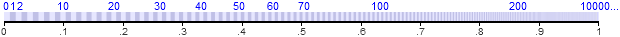If you were to throw a dart at this number line, the likelihood of hitting each partition is about the same as the likelihood of encountering each delta value in one of our sorted sequences.

That’s exactly the kind of information that’s useful for arithmetic coding.

## The Idea Behind Arithmetic Encoding

Arithmetic encoding is able to encode a sequence of elements – in this case, delta values – by progressively subdividing the real number line into finer and finer partitions. At each step, the relative width of each partition is determined by the probability of encountering each element.

Suppose the first delta value in the sequence is 27. We begin by locating the corresponding partition in the original number line, and zooming into it. This gives us a new interval of the real number line to work with – in this case, roughly from .236 to .243 – which we can then subdivide further. Let’s use the same proportions we used for the first element.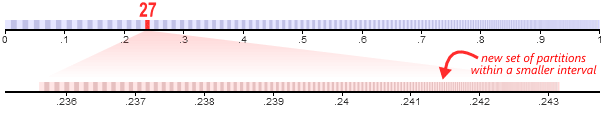Suppose the next element in the sequence is 39. Again, we locate the corresponding partition and zoom in, subdividing the interval into even finer partitions.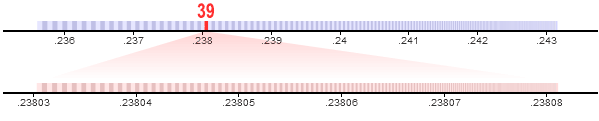In this way, the interval gets progressively smaller and smaller. We repeat these steps one million times: once for each element in the sequence. After that, all we need to store is a single real value which lies somewhere within the final partition. This value will unambiguously identify the entire one-million-element sequence.

As you can imagine, to represent this value, it’s going to take a lot of precision. Hundreds of thousands times more precision than you’ll find in any single- or even double-precision floating-point value. What we need is a way to represent a fractional value having millions of significant digits. And in arithmetic coding, that’s exactly what the final encoded bit stream is. It’s one giant binary fraction having millions of binary digits, pinpointing a specific value somewhere within the interval [0, 1) with laser precision.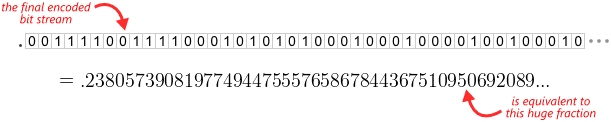That’s great, you might be thinking, but how the heck do we even work with numbers that precise?

This post has already become quite long, so I’ll answer that question in a separate post. You don’t even have to wait, because it’s already published: See Arithmetic Encoding Using Fixed-Point Math for the thrilling conclusion!